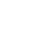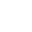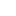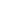# Prim’s – Minimum Spanning Tree (MST) |using Adjacency List and Priority Queue with decrease key

Earlier we have seen what is Prim’s algorithm is and how it works. In this article we will see its implementation using adjacency list and Priority Queue.

We strongly recommend to read – prim’s algorithm and implementation of priority queue.

Example:Implementation – Adjacency List and Priority Queue with decrease key

In last article we discussed the implementation using min-heap. In this article our approach will be same except in place of min-heap we will use priority queue with decrease-key function. This approach will have more time complexity which we will improve in the next article – priority queue – better approach.

1. Create priority queue of size = no of vertices.
2. Create a heapNode for each vertex which will store two information’s.
1. Vertex
2. key
3. Use inPriorityQueue[] to keep track of the vertices which are currently in priority queue.
4. Create key[] to keep track of key value for each vertex. (keep updating it as heapNode key for each vertex)
5. For each heapNode, initialize key as MAX_VAL except the heapNode for the first vertex for which key will 0. (Start from first vertex).
6. Override the Comparator of priority queue to sort them based on the key. Insert all the heapNodes into priority queue. inPriorityQueue [v] = true for all vertices.
7. while priority queue is not empty
1. Extract the min node from the priority queue, say it vertex u and add it to the MST.
2. Decrease key: Iterate through all the adjacent vertices of above vertex u and if adjacent vertex(say it’s v) is still part of inPriorityQueue [] (means not in MST) and key of vertex v> u-v weight then update the node in priority queue. Find the node for vertex v in priority queue, remove it, and update it as key of vertex v = u-v weight and insert it again. This step increases the complexity of this implementation.
8. We will use Result object to store the result of each vertex. Result object will store 2 information’s.
1. First the parent vertex means from which vertex you can visit this vertex. Example if for vertex v, you have included edge u-v in mst[] then vertex u will be the parent vertex.
2. Second weight of edge u-v. If you add all these weights for all the vertices in mst[]  then you will get Minimum spanning tree weight.

Time Complexity:

Total vertices: V, Total Edges : E

• O(logV) – to extract each vertex from queue. So for V vertices – O(VlogV)
• O(V) – each time decrease the key value of a vertex. Decrease key will be called for at most once for each edge. So for total E edge – O(EV)
• So over all complexity: O(VlogV) + O(EVlogV) = O(EVlogV)

See the animation below for more understandingComplete Code:

Output:

```Minimum Spanning Tree:

Edge: 1 - 2 key: 1
Edge: 2 - 0 key: 3
Edge: 3 - 1 key: 2
Edge: 4 - 3 key: 2
Edge: 5 - 4 key: 6
Total minimum key: 14
```

__________________________________________________
Top Companies Interview Questions..-

If you find anything incorrect or you feel that there is any better approach to solve the above problem, please write comment.
__________________________________________________